Courses

# Competition Level Test: Electromagnetic Induction

## 25 Questions MCQ Test Physics For JEE | Competition Level Test: Electromagnetic Induction

Description
This mock test of Competition Level Test: Electromagnetic Induction for Class 12 helps you for every Class 12 entrance exam. This contains 25 Multiple Choice Questions for Class 12 Competition Level Test: Electromagnetic Induction (mcq) to study with solutions a complete question bank. The solved questions answers in this Competition Level Test: Electromagnetic Induction quiz give you a good mix of easy questions and tough questions. Class 12 students definitely take this Competition Level Test: Electromagnetic Induction exercise for a better result in the exam. You can find other Competition Level Test: Electromagnetic Induction extra questions, long questions & short questions for Class 12 on EduRev as well by searching above.
QUESTION: 1

Solution:
QUESTION: 2

Solution:
QUESTION: 3

### An electric current i1 can flow either direction through loop (1) and induced current i2 in loop (2). Positive i1 is when current is from `a' to `b' in loop (1) and positive i2 is when the current is from `c' to `d' in loop (2)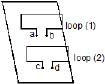In an experiment, the graph of i2 against time `t' is as shown below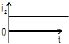Which one(s) of the following graphs could have caused i2 to behave as give above.

Solution:

ϕ2=Mi1⇒i2=(1/R)(−dϕ2/dt)
i2=−MdI1/Rdt

QUESTION: 4

Figure shows a bar magnet and a long straight wire W, carrying current into the plane of paper. Point P is the point of intersection of axis of magnet and the line of shortest distance between magnet and the wire. If p is the midpoint of the magnet, then which of the following statements is correct ?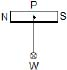Solution:

There is a long straight wire W, the current flowing direction in the wire is into the plane of paper and the point of intersection on the axis of magnet is P.
If the midpoint of the bar magnet is P then the distance from the point P to the wire will be the shortest distance.
So, the bar magnet will experience a force along the line of shortest distance.

QUESTION: 5

A negative charge is given to a nonconducting loop and the loop is rotated in the plane of paper about its centre as shown in figure. The magnetic field produced by the ring affects a small magnet placed above the ring in the same plane :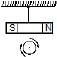Solution:
*Multiple options can be correct
QUESTION: 6

Figure shown plane figure made of a conductor located in a magnetic field along the inward normal to the plane of the figure. The magnetic field starts diminishing. Then the induced current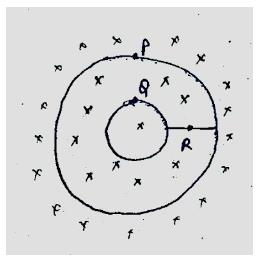Solution:As the field diminishes the current is induced in the coils such that if opposes the diminishing of the field. Hence, current is induced in clockwise directing in both the loops.
And as the loops are closed no current will come out of the loops and no current at point R.
Hence option A C D is the correct option.

*Multiple options can be correct
QUESTION: 7

A conducting wire frame is placed in a magnetic field which is directed into the paper. The magnetic field is increasing at a constant rate. The directions of induced currents in wires AB and CD are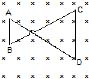Solution:

Consider the surface facing upwards having points A, B, C and D in anticlockwise direction when viewing towards the surface from above. Let this be S
flux = S×B
As the flux increases, by Lenz's Law the induced current will be in the direction C B A D C, so as to oppose the increase in flux.

*Multiple options can be correct
QUESTION: 8

A bar magnet is moved along the axis of copper ring placed far away from the magnet. Looking from the side of the magnet, an anticlockwise current is found to be induced in the ring. Which of the following may be true?

Solution:

When magnet is moving towards the ring, as the current flows in anti-clockwise direction when seen from magnet, a magnetic field directed towards the magnet from the ring is observed.
By Lenz's law this field is to oppose the movement of the magnet towards the ring.
Therefore the N-pole should be facing the ring, as only then will the induced field by the ring will oppose the movement of the approaching magnet.
Therefore, the north pole faces the ring and the magnet moves towards it  and the  south pole faces the ring and the magnet moves away from it.

*Multiple options can be correct
QUESTION: 9

Two circular coils P & Q are fixed coaxially & carry currents I1 and Irespectively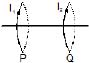Solution:
*Multiple options can be correct
QUESTION: 10

Which of the following quantities can be written in SI units in K gm2 A-2 S-3 ?

Solution:

The dimensional formula of a given unit is [ML2T−3A−2].
So, from the given option only resistance has this dimensional formula so resistance is the correct answer.

*Multiple options can be correct
QUESTION: 11

AB and CD are smooth parallel rails, separated by a distance l, and inclined to the horizontal at an angle q. A uniform magnetic field of magnitude B, directed vertically upwards, exists in the region. EF is a conductor of mass m, carrying a current i. For EF to be in equilibrium,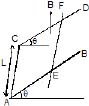Solution:

Force on EF,
Fmag​​=i∫(dl×B)=iLBsin(90−θ)=iLBcosθ
Fmag​​ up the inclined plane.
Component of weight of EF down the inclined plane: mgsinθ
For EF to be in equilibrium, iLBcosθ=mgsinθ
∴iLB=mgtanθ
For Fmag​ to be up the inclined plane, it needs to flow from E to F.

*Multiple options can be correct
QUESTION: 12

AB and CD are smooth parallel rails, separated by a distance l, and inclined to the horizontal at an angle q. A uniform magnetic field of magnitude B, directed vertically upwards, exists in the region. EF is a conductor of mass m, carrying a current i. if B is normal to the plane of the railsSolution:

As seen from the image, in the direction of magnetic field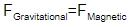mgsinθ=il×B
mgsinθ=ilB

*Multiple options can be correct
QUESTION: 13

A conducting rod PQ of length L = 1.0 m is moving with a uniform speed v = 20 m/s in a uniform magnetic field B = 4.0 T directed into the paper. A capacitor of capacity C = 10 mF is connected as shown in figure. Then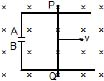Solution:

According to Fleming’s right-hand rule induced current flows from Q to P.
Hence P is at higher potential and Q is at lower potential.
Therefore, A is positively charged and B is negatively charged.
Hence:
qA​=+800μC and qb​=−800μC

*Multiple options can be correct
QUESTION: 14

two different arrangements in which two square wire frames of same resistance are placed in a uniform constantly decreasing magnetic field B.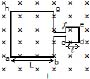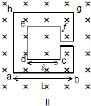The value of magnetic flux in each case is given by

Solution:

f=BA, area of square=(side)2
so, for case I.
loops is continuous,
f=(L2+l2)B
for case II
loop is opposite,
so, flux should be subtracted
f=(L2-l2)B

QUESTION: 15

Two different arrangements in which two square wire frames of same resistance are placed in a uniform constantly decreasing magnetic field B.The direction of induced current in the case I is

Solution:
*Multiple options can be correct
QUESTION: 16

Two different arrangements in which two square wire frames of same resistance are placed in a uniform constantly decreasing magnetic field B.The direction of induced current in the case II is

Solution: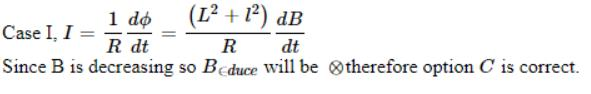*Multiple options can be correct
QUESTION: 17

Two different arrangements in which two square wire frames of same resistance are placed in a uniform constantly decreasing magnetic field B.Solution:
*Multiple options can be correct
QUESTION: 18

A conducting ring of radius a is rotated about a point O on its periphery as shown in the figure in a plane perpendicular to uniform magnetic field B which exists everywhere. The rotational velocity is w.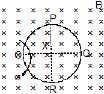Choose the correct statement(s) related to the potential of the points P, Q and R

Solution:
*Multiple options can be correct
QUESTION: 19

A conducting ring of radius a is rotated about a point O on its periphery as shown in the figure in a plane perpendicular to uniform magnetic field B which exists everywhere. The rotational velocity is w.Solution:
*Multiple options can be correct
QUESTION: 20

A conducting ring of radius a is rotated about a point O on its periphery as shown in the figure in a plane perpendicular to uniform magnetic field B which exists everywhere. The rotational velocity is w.Choose the correct statement(s) related to the induced current in ring

Solution:

In the given question neither the magnetic field nor the area of the ring is changing. So there will be a constant flux in the ring and hence there will be no EMF (or) current induced in the ring.

*Answer can only contain numeric values
QUESTION: 21

The horizontal component of the earth's magnetic field at a place is 3 × 10-4 T and the dip is tan-1(4/3). A metal rod of length 0.25 m placed in the north-south position is moved at a constant speed of 10 cm/s towards the east. Find the e.m.f induced in the rod.

Solution:
QUESTION: 22

A wire forming one cycle of sine curve is moved in x-y plane with velocity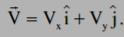. There exist a magnetic field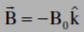. Find the motional emf develop across the ends PQ of wire.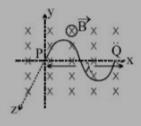Solution:
*Answer can only contain numeric values
QUESTION: 23

A conducting circular loop is placed in a uniform magnetic field of 0.02 T, with its plane perpendicular to the field. If the radius of the loop starts shrinking at a constant rate of 1.0 mm/s, then find the emf induced in the loop, at the instant when the radius is 4 cm.

Solution:

Induced EMF E=− (dϕ /dt) ​, ϕ=A.B, A=πR2
this gives, E=−B (dA /dt) ​=−πB×2R (dR/dt) ​
=π×0.02×2×0.04×0.001
=16π×10−7V

QUESTION: 24

Find the dimension of the quantity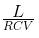, where symbols have usual meaning.

Solution:

We know that:
[V]=[iR]=[L (di/dt) ​]
[i][R]= [L][i]​/ [t]
Thus, [L/R​]=[t]=T
[L]= [V][t]​/[i]
Also, i=C (dV/dt) ​, or [CV]=[i][t]=AT
Thus, [L/RCV​]=T/AT=A−1

*Answer can only contain numeric values
QUESTION: 25

A rectangular loop with a sliding connector of length l = 1.0 m is situated in a uniform magnetic field B = 2T perpendicular to the plane of loop. Resistance of connector is r = 2W. Two resistances of 6W and 3W are connected as shown in figure. Find the external force required to keep the connector moving with a constant velocity v = 2m/s.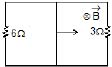Solution:

6Ω and 3Ω resistors are in parallel and equivalent resistance is 2Ω+2Ω=4Ω.
F=Bil=BL (​BLv​/Req)
= ​B2l2v/Req ​=(22×12×2​)/4=2N